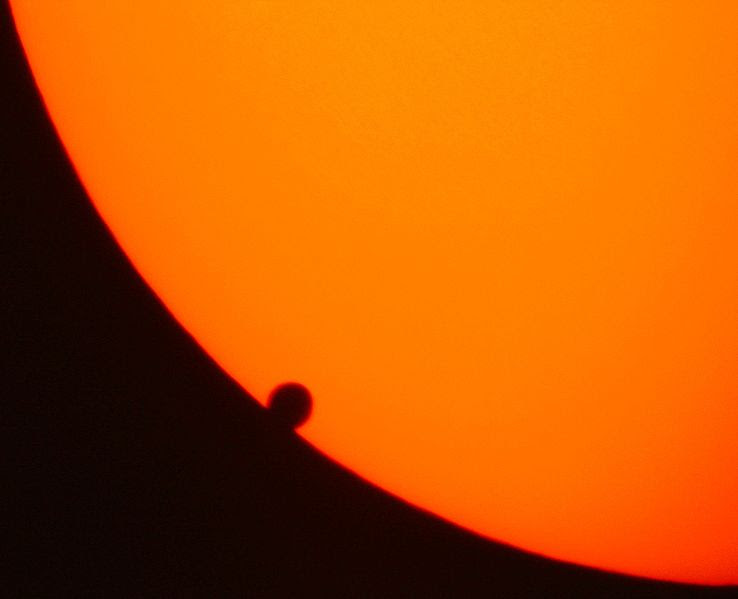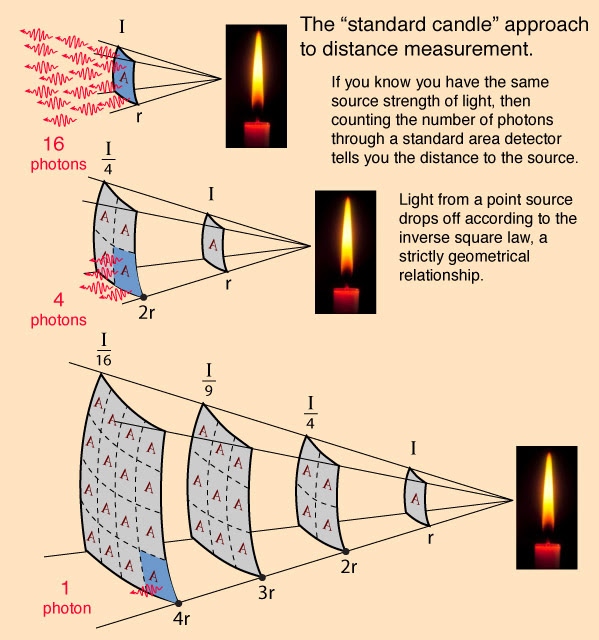## Friday, February 19, 2021

### How do we measure the distances to things in space?

It is not an easy thing to measure the distances to objects in the universe since these objects are usually very far away. We can't just run out there with a ruler! To measure distances in the universe, we will need to construct what is commonly referred to as a "cosmic distance ladder". In other words, astronomers use different methods to determine the distances to objects; the
specific method which is used depends on how far away the object.

The cosmic distance ladder (also known as the extragalactic distance scale) is the succession of methods by which astronomers determine the distances to celestial objects. A real direct distance measurement of an astronomical object is possible only for those objects that are close enough (within about a thousand parsecs) to Earth. The techniques for determining distances to more distant objects are all based on various measured correlations between methods that work at close distances with methods that work at larger distances. Several methods rely on a standard candle, which is an astronomical object that has a known luminosity.

The ladder analogy arises because no one technique can measure distances at all ranges encountered in astronomy. Instead, one method can be used to measure nearby distances, a second can be used to measure nearby to intermediate distances, and so on. Each rung of the ladder provides information that can be used to determine the distances at the next higher rung.

The Astronomical Unit (AU)

An astronomical unit (abbreviated as au) is a unit of length now defined as exactly 149,597,870,700m (92,955,807.3 mi), or roughly the average Earth–Sun distance. Historically, observations of transits of Venus were crucial in determining the AU; in the first half of the 20th Century, observations of asteroids were also important. Presently the AU is determined with high precision using radar measurements of Venus and other nearby planets and asteroids, and by tracking interplanetary spacecraft in their orbits around the Sun through the Solar System. Kepler’s Laws provide precise ratios of the sizes of the orbits of objects revolving around the Sun, but not a real measure of the orbits themselves. Radar provides a value in kilometers for the difference in two orbits’ sizes, and from that and the ratio of the two orbit sizes, the size of Earth’s orbit comes directly.Transits of Venus across the face of the Sun were, for a long time, the best method of measuring the astronomical unit, despite the difficulties and the rarity of observations. Source: NASA

Parallax

The most important fundamental distance measurements come from trigonometric parallax. As the Earth orbits around the Sun, the position of nearby stars will appear to shift slightly against the more distant background. These shifts are angles in a right triangle, with 2 AU making the short leg of the triangle and the distance to the star being the long leg. The amount of shift is quite small, measuring 1 arcsecond for an object at a distance of 1 parsec (3.26 light-years), thereafter decreasing in angular amount as the reciprocal of the distance. Astronomers usually express distances in units of parsecs; light-years are used in popular media, but almost invariably values in light-years have been converted from numbers tabulated in parsecs in the original source.

Because parallax becomes smaller for a greater stellar distance, useful distances can be measured only for stars whose parallax is larger than the precision of the measurement. Parallax measurements typically have an accuracy measured in milliarcseconds. In the 1990s, for example, the Hipparcos mission obtained parallaxes for over a hundred thousand stars with a precision of about a milliarcsecond, providing useful distances for stars out to a few hundred parsecs.Using Parallax to measure a star as seen from earth 6 months aparts. Credit: ESA Science & Technology:

Standard Candle

A standard candle is an astronomical object that has a known absolute magnitude. They are extremely important to astronomers since by measuring the apparent magnitude of the object we can determine its distance using the formula:

m-M = 5 log d – 5

where m is the apparent magnitude of the object, M is the absolute magnitude of the object, and d is the distance to the object in parsecs.

The most commonly used standard candles in astronomy are Cepheid Variable stars and RR Lyrae stars. In both cases, the absolute magnitude of the star can be determined from its variability period.The Standard Candle approach to measuring distance. Credit: Univ. of California

Intergalactic Measurements
Distances from the Earth to nearby stars can conveniently be expressed in parsecs; for example, the nearest star, Proxima Centauri, is 1.295 parsecs distant. Because a parsec equals 3.27 light years, that's 4.225 light years. Even parsecs, however, prove inadequate for measuring distances within the galaxy or intergalactic distances. Astrophysicists frequently express these in kiloparsecs and megaparsecs, which equal 1,000 and 1 million parsecs, respectively. For example, the center of the galaxy is about 8 kiloparsecs distant, which equals 8,000 parsecs, or 26,160 light years. You’d need 16 digits to express that number with kilometers or miles.